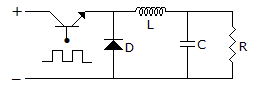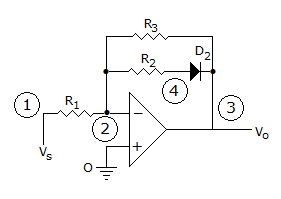# Electronics and Communication Engineering - Analog Electronics

41.

Assertion (A): In the circuit shown figure the diode is used to protect the active devices. Transistor T goes into saturation when a positive pulse is applied at base and non conducting

Reason (R): When the current in an inductor is suddenly stopped, a high voltage is induced across it.A. Both A and R are correct and R is correct explanation for A B. Both A and R are correct but R is not correct explanation for A C. A is correct R is wrong D. A is wrong R is correct

Explanation:

No answer description available for this question. Let us discuss.

42.

When the ac input signal is very large the instantaneous operating point CE amplifier moves all the way between

 A. ac saturation and ac cut off points B. ac operating and ac cut off points C. ac saturation and ac operating points D. none of the above

Explanation:

No answer description available for this question. Let us discuss.

43.

For full wave rectification four diode bridge rectifier is claimed to have following advantages:

1. Less expensive transformer
2. Small size transformer
3. Suitable for higher voltage applications
Which of these are correct?

 A. 1 and 2 only B. 1 and 3 only C. 2 and 2 only D. 1, 2, 3

Explanation:

No answer description available for this question. Let us discuss.

44.

Following figure is a signal conditioning Amplifier, changes gain depending upon the polarity of Vs. What will be circuit voltage gain for + Vs and for - Vs if D2 is ideal.A. AV = -R3/R1 B. R3/R1 C. R1/R3 D. 1 + R3/R1

Explanation:

No answer description available for this question. Let us discuss.

45.

Which class of amplifiers operates with least distortion?

 A. Class A B. Class B C. Class C D. Class AB